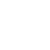Home > Science & Mathematics > Mathematics > Calculus & mathematical analysis > Equilibrium States in Ergodic Theory
3%# Equilibrium States in Ergodic Theory

•••••International Edition

This book provides a detailed introduction to the ergodic theory of equilibrium states giving equal weight to two of its most important applications, namely to equilibrium statistical mechanics on lattices and to (time discrete) dynamical systems. It starts with a chapter on equilibrium states on finite probability spaces that introduces the main examples for the theory on an elementary level. After two chapters on abstract ergodic theory and entropy, equilibrium states and variational principles on compact metric spaces are introduced, emphasizing their convex geometric interpretation. Stationary Gibbs measures, large deviations, the Ising model with external field, Markov measures, Sinai-Bowen-Ruelle measures for interval maps and dimension maximal measures for iterated function systems are the topics to which the general theory is applied in the last part of the book. The text is self contained except for some measure theoretic prerequisites that are listed (with references to the literature) in an appendix.

## Best Sellers

Product Details
• ISBN-13: 9780521595346
• Publisher: Cambridge University Press
• Publisher Imprint: Cambridge University Press
• Depth: 6
• Language: English
• Returnable: N
• Spine Width: 11 mm
• Width: 153 mm
• ISBN-10: 0521595347
• Publisher Date: 22 Jan 1998
• Binding: Paperback
• Height: 227 mm
• No of Pages: 192
• Series Title: London Mathematical Society Student Texts
• Weight: 290 gr

## Similar Products

Write A Review
Write your own book review for Equilibrium States in Ergodic Theory
•••••Top Reviews
Be the first to write a review on this book Equilibrium States in Ergodic Theory